Maths-
General
Easy

Question

# A fort had enough food for 80 soldiers for 60 days. How long would the food last if 20 more soldiers join after 15 days?Hint:

## The correct answer is: 15 days.

### Let the number of days be represented as x and the number of soldiers be represented as y. The number of days will decrease if the number of soldiers are increased so we can conclude that y is in inverse relationship with x. Let’s say the proportional relationship is given asy =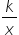……..(1)Step 1 of 2:After 15 days, the food is sufficient for 80 soldiers for (60 – 15) days = 45 days. So,  x = 45 and y = 80. Putting the values in equation (1)80 =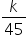k = 80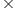45  = 3600Step 2 of 2:Now we are asked to find the number of days the food will last if 20 more soldiers will join after 15 days. So, the total number of students becomes 100. Let the number of days be “d”. So, x = d and y = 100. Putting the values in equation (1)100 =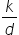Now, put the value of k = 3600d =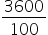d = 36 daysFinal Answer: Hence, the food will last for 36 days if 20 more soldiers join after 15 days.#### With Turito Foundation.#### Get an Expert Advice From Turito.